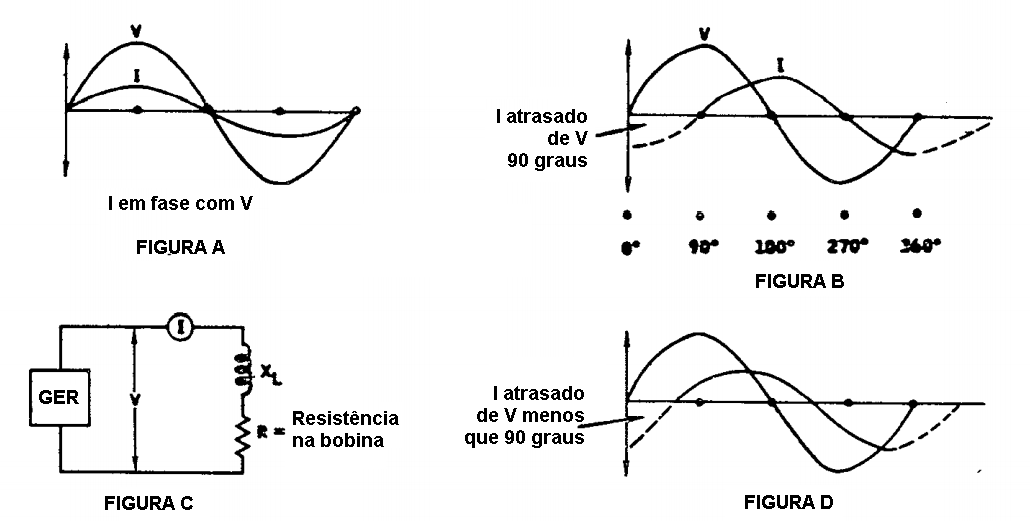PB_00 - Capítulo 5 - Análise de Fase - Página 5-035When a resistor is connected across a generator, the current (I) will be in phase with the voltage (V) as shown in view A. If the resistor is now replaced by a coil, the current will no"longer be in phase with the voltage. The coil as you have seen has both resistance and inductance (L). The inductance in turn is expressed as the inductive reactance (XL) which you have learned is determined by the inductance (L) and by the frequency applied to the coil. (XL = 6.28 f).

In some cases, the inductive reactance (XL) is so much greater than the coil's resistance that we can disregard the resistance. Under these conditions, it can be shown that the current (I) lags the voltage (V) by 90 degrees as shown in view B. It can also be shown that when the resistance is a significant value (views C and D) the current will lag the voltage by a value less than 90 degrees. The current lag shown in view D reresents the effect of both the coil's inductance reactance and the resistance. A change in either value will change the lag between the current and the voltage.

If a change in the coil's magnetic field is made by the presence of a specimen, will the lag between the current and the voltage:

...... Change.

...... Remain the same.